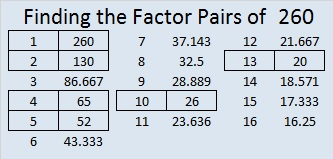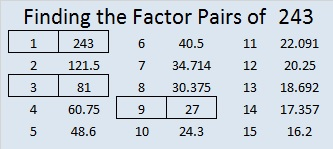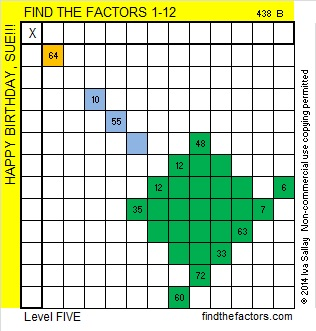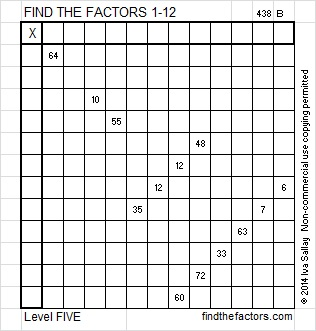# 260 Some Thoughts on Those Four Consecutive Numbers

• 260 is a composite number.
• Prime factorization: 260 = 2 x 2 x 5 x 13, which can be written 260 = (2^2) x 5 x 13
• The exponents in the prime factorization are 2, 1 and 1. Adding one to each and multiplying we get (2 + 1)(1 + 1)(1 + 1) = 3 x 2 x 2 = 12. Therefore 260 has 12 factors.
• Factors of 260: 1, 2, 4, 5, 10, 13, 20, 26, 52, 65, 130, 260
• Factor pairs: 260 = 1 x 260, 2 x 130, 4 x 65, 5 x 52, 10 x 26, or 13 x 20
• Taking the factor pair with the largest square number factor, we get √260 = (√4)(√65) = 2√65 ≈ 16.125Recently I wrote about the smallest four-consecutive-numbers whose square roots could all be simplified. The same numbers were also the smallest four consecutive numbers to have the same number of factors.Each of those numbers had 6 factors, and guess what, ANY number with exactly 6 factors can have its square root simplified. The prime factorization of ANY number with exactly 6 factors can be expressed in one of the three following ways:Since numbers with six factors always have a prime factor raised to a power greater than one, they can always have their square roots simplified. The fact that those four consecutive numbers have the same number of factors makes them extraordinary; that they all can have their square roots simplified is merely the natural consequence of that extraordinary fact.

# 245 – The Last of Four Consecutive Numbers

• 245 is a composite number.
• Prime factorization: 245 = 5 x 7 x 7, which can be written 245 = 5 x (7^2)
• The exponents in the prime factorization are 1 and 2. Adding one  to each and multiplying we get (1 + 1)(2 + 1) = 2 x 3 = 6. Therefore 245 has 6 factors.
• Factors of 245: 1, 5, 7, 35, 49, 245
• Factor pairs: 245 = 1 x 245, 5 x 49, or 7 x 35
• Taking the factor pair with the largest square number factor, we get √245 = (√5)(√49) = 7√5 ≈ 15.652I was surprised when I noticed that the square roots of these 4 consecutive numbers – 242, 243, 244, and 245 could all be simplified.

The square root of a whole number can only be simplified if that whole number has a square number as one of its factors. All four of these numbers meet that condition, and they are the first four consecutive numbers to do so.

For numbers less than or equal to 240, there are only 3 sets of 3 consecutive square roots that can be simplified.

• √48 = 4√3
• √49 = 7
• √50 = 5√2
• √98 = 7√2
• √99 = 3√11
• √100 = 10
• √124 = 2√31
• √125 = 5√5
• √126 = 3√14

242, 243, 244, and 245 also have another distinction. They each have exactly 6 factors and are the smallest consecutive four numbers to have the same number of factors.

# 243 and Level 5

• 243 is a composite number.
• Prime factorization: 243 = 3 x 3 x 3 x 3 x 3, which can be written 243 = (3^5)
• The exponent in the prime factorization is 5. Adding one we get (5 + 1) = 6. Therefore 243 has 6 factors.
• Factors of 243: 1, 3, 9, 27, 81, 243
• Factor pairs: 243 = 1 x 243, 3 x 81, or 9 x 27
• Taking the factor pair with the largest square number factor, we get √243 = (√3)(√81) = 9√3 ≈ 15.588This puzzle is for my sister, who is great at completing these Level 5 puzzles. Here is a black and white version for those who may prefer it:Print the puzzles or type the factors on this excel file: 12 Factors 2014-09-22